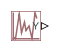# PS Uniform Random Number

Generate uniformly distributed random numbers for physical modeling

•Libraries:
Simscape / Foundation Library / Physical Signals / Sources

## Description

The PS Random Number block generates uniformly distributed random numbers over the interval you specify. To generate normally (Gaussian) distributed random numbers, use the PS Random Number block.

The block behavior is the same as the Simulink® Uniform Random Number block (except that it generates a physical signal rather than a Simulink signal).

You have an option to specify an initial time offset as part of the Sample time parameter. In this case, the block outputs 0 until the simulation time reaches the `offset` value, at which point the random sequence starts.

PS Uniform Random Number blocks that use the same seed and parameters generate a repeatable sequence. The seed resets to the specified value each time a simulation starts.

## Ports

### Output

expand all

Output physical signal.

## Parameters

expand all

The minimum output value generated by the block.

The maximum output value generated by the block.

The starting seed for the random number generator. Output is repeatable for a given seed. The seed must be an integer in the range of 0 to (2^32 – 1).

The value of the time `step` interval. The default `step` value is `1` s. To specify an initial time offset, enter the parameter value as ```[step, offset]```, otherwise the `offset` value is assumed to be `0`. The offset must be less than the step size.

## Version History

Introduced in R2013a

expand all